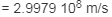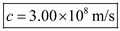# The speed of light to five significant figures is 2.9979 x 108 m/s. What is the speed of light

The speed of light to five significant figures is 2.9979 x 108 m/s. What is the speed of light to three significant figures?

Speed of light c